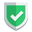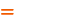我们相信：世界是美好的，你是我也是。平行空间的世界里面，不同版本的生活也在继续...

## 测试代码

``````import pathlib
f1 = pathlib.Path("./dir/").glob('*')
f2 = pathlib.Path("./").glob('dir/*')``````
``````f3 = pathlib.Path("./dir/").glob('**')
f4 = pathlib.Path("./").glob('dir/**')``````
``````f5 = pathlib.Path("./dir/").glob('*.*')
f6 = pathlib.Path("./").glob('dir/*.*')``````

``````f7 = pathlib.Path("./dir/").glob('星/s?.txt')
f8 = pathlib.Path("./dir/").glob('星星/s?.txt')
f9 = pathlib.Path("./dir/").glob('星.星/s?.txt')``````

``````{WindowsPath('dir/sub/s2.txt')}
{WindowsPath('dir/sub/s2.txt'), WindowsPath('dir/sub/subsub/s2.txt'), WindowsPath('dir/s2.txt')}
set()``````

## 输出结果

`glob.glob()``list`
`glob.iglob()``generator`
`pathlib.Path('./').glob()``generator`

``````import glob
import pathlib

f10 = glob.iglob('dir/s?.txt')
f11 = pathlib.Path("./dir/").glob('星/s?.txt')

print(list(f10))  # {'dir\\s2.txt'}
print(list(f11))  # {WindowsPath('dir/sub/s2.txt')}

f10 = glob.iglob('dir/s?.txt')
f11 = pathlib.Path("./dir/").glob('星/s?.txt')

print([f for f in f10])  # {'dir\\s2.txt'}
print([f for f in f11])  # {WindowsPath('dir/sub/s2.txt')}``````

• 两者对于路径的表述输出，也是不一样的。
• 两者都是迭代器类型的，所以只能访问一次。

## `pathlib.`对比`glob.`

`pathlib`模块下的`glob()`

• 只需要填写路径信息即可，不需要参数`recursive`
• 路径信息中，可以像以前一样传递，也可以分开写。
• 结果输出是个迭代器类型。

glob.glob()`dir/**`默认`false`glob.glob("dir/**",recursive=True)
pathlib.Path("./").glob()`dir/**`不需要pathlib.Path("./").glob("dir/**")

`*`当前所有文件及文件夹(含"点文件")当前目录所有文件及文件夹
`**`所有的目录，不包括文件`recursive=true`时，所有目录及文件(不含"点文件")
`*.*`当前目录下的所有文件(含"点文件")当前目录下的所有文件(不含"点文件")

## 总结

• 使用`pathlib`模块下的`glob()` 对比 `glob`模块下面的`glob()`，前者的结果更加合理一些，因为输出哪些特殊文件，但是写法上不如`glob()`下更简单一些。推荐使用：`pathlib.Path('./').glob()`
• 对于`**`的解释，两者差别巨大，请注意区分。
• https://newsn.net/tag/python/如果本文对您有帮助，或者节约了您的时间，欢迎打赏瓶饮料，建立下友谊关系。本博客不欢迎：各种镜像采集行为。请尊重原创文章内容，转载请保留作者链接。【福利】 腾讯云最新爆款活动！1核2G云服务器首年50元！【源码】本文代码片段及相关软件，请点此获取更多信息【绝密】秘籍文章入口，仅传授于有缘之人python